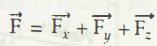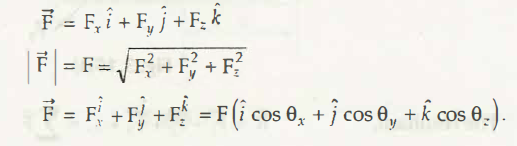F x =F cos θ x

F y = F cos θ y

F z = F cos θ zThus these are the unit vectors of the points x, y, and z directions respectively.

Therefore, θx, θ y, θ z are the angles made by F with the axis x, y, and z(the rest is referred to the chapter vector algebra)

Links of Previous Main Topic:-

Links of Next Mechanical Engineering Topics:-

### Submit Your Assignment### Customer Reviews

My Homework Help
Rated 5.0 out of 5 based on 510 customer reviews at